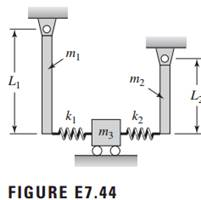### Create an Account

Home / Questions / Consider the coupled pendulum system shown in Figure E744 a Use Lagrange’s equations to d...

# Consider the coupled pendulum system shown in Figure E744 a Use Lagrange’s equations to derive the equations of motion. Include the effects of gravity Put the results in matrix form

Consider the coupled pendulum system shown in Figure E7.44.

a) Use Lagrange’s equations to derive the equations of motion. Include the effects of gravity. Put the results in matrix form.

b) For m1 = 10 kg, m2= 15 kg, m3 = 5 kg, k1 = k2 = 100 N/m, L1 = 0.5 m, and L2 = 0.4 m, determine the natural frequencies and mode shapes of the system.May 16 2020 View more View LessSubscribe To Get Solution